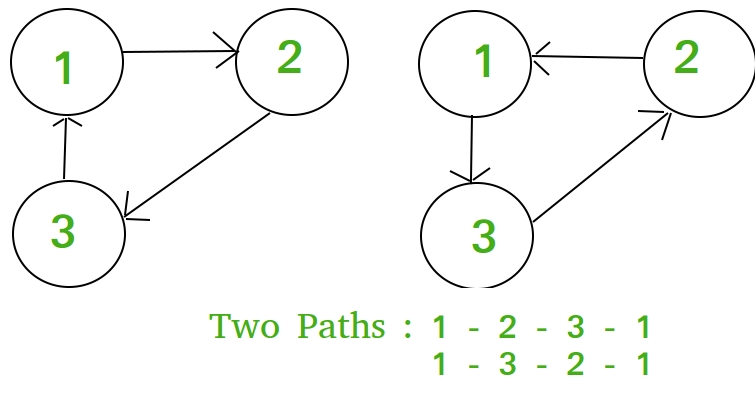# Number of loops of size k starting from a specific node

Given two positive integer n, k. Consider an undirected complete connected graph of n nodes in a complete connected graph. The task is to calculate the number of ways in which one can start from any node and return to it by visiting K nodes.

Examples:

Input : n = 3, k = 3
Output : 2Input : n = 4, k = 2
Output : 3


## Recommended: Please try your approach on {IDE} first, before moving on to the solution.

Lets f(n, k) be a function which return number of ways in which one can start from any node and return to it by visiting K nodes.
If we start and end from one node, then we have K – 1 choices to make for the intermediate nodes since we have already chosen one node in the beginning. For each intermediate choice, you have n – 1 options. So, this will yield (n – 1)k – 1 but then we have to remove all the choices cause smaller loops, so we subtract f(n, k – 1).
So, recurrence relation becomes,
f(n, k) = (n – 1)k – 1 – f(n, k – 1) with base case f(n, 2) = n – 1.
On expanding,
f(n, k) = (n – 1)k – 1 – (n – 1)k – 2 + (n – 1)k – 3 ….. (n – 1) (say eqn 1)

Dividing f(n, k) by (n – 1),
f(n, k)/(n – 1) = (n – 1)k – 2 – (n – 1)k – 3 + (n – 1)k – 4 ….. 1 (say eqn 2)

On adding eqn 1 and eqn 2,
f(n, k) + f(n, k)/(n – 1) = (n – 1)k – 1 + (-1)k
f(n, k) * ( (n -1) + 1 )/(n – 1) = (n – 1)k – 1 + (-1)kBelow is the implementation of this approach:

## C++

 // C++ Program to find number of cycles of length  // k in a graph with n nodes.  #include  using namespace std;     // Return the Number of ways from a  // node to make a loop of size K in undirected  // complete connected graph of N nodes  int numOfways(int n, int k)  {      int p = 1;         if (k % 2)          p = -1;         return (pow(n - 1, k) + p * (n - 1)) / n;  }     // Driven Program  int main()  {      int n = 4, k = 2;      cout << numOfways(n, k) << endl;      return 0;  }

## Java

 // Java Program to find number of  // cycles of length k in a graph  // with n nodes.  public class GFG {             // Return the Number of ways      // from a node to make a loop      // of size K in undirected      // complete connected graph of      // N nodes      static int numOfways(int n, int k)      {          int p = 1;                 if (k % 2 != 0)              p = -1;                 return (int)(Math.pow(n - 1, k)                      + p * (n - 1)) / n;      }             // Driver code      public static void main(String args[])      {          int n = 4, k = 2;                 System.out.println(numOfways(n, k));      }  }     // This code is contributed by Sam007.

## Python3

 # python Program to find number of   # cycles of length k in a graph   # with n nodes.     # Return the Number of ways from a  # node to make a loop of size K in  # undirected complete connected   # graph of N nodes  def numOfways(n,k):             p = 1        if (k % 2):          p = -1        return (pow(n - 1, k) +                    p * (n - 1)) / n     # Driver code  n = 4 k = 2 print (numOfways(n, k))     # This code is contributed by Sam007.

## C#

 // C# Program to find number of cycles  // of length k in a graph with n nodes.  using System;     class GFG {             // Return the Number of ways from      // a node to make a loop of size      // K in undirected complete       // connected graph of N nodes      static int numOfways(int n, int k)      {          int p = 1;                 if (k % 2 != 0)              p = -1;                 return (int)(Math.Pow(n - 1, k)                       + p * (n - 1)) / n;      }             // Driver code      static void Main()      {          int n = 4, k = 2;                     Console.Write( numOfways(n, k) );      }  }     // This code is contributed by Sam007.

## PHP

 

Output:

3


Attention reader! Don’t stop learning now. Get hold of all the important DSA concepts with the DSA Self Paced Course at a student-friendly price and become industry ready.

My Personal Notes arrow_drop_upCheck out this Author's contributed articles.

If you like GeeksforGeeks and would like to contribute, you can also write an article using contribute.geeksforgeeks.org or mail your article to contribute@geeksforgeeks.org. See your article appearing on the GeeksforGeeks main page and help other Geeks.

Please Improve this article if you find anything incorrect by clicking on the "Improve Article" button below.

Improved By : Sam007, vt_m